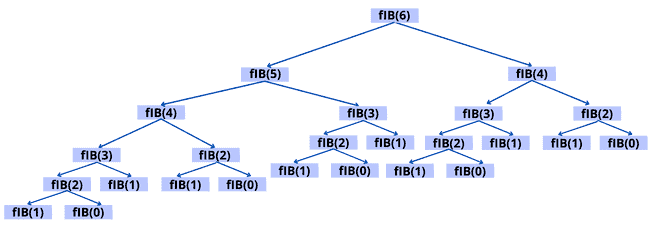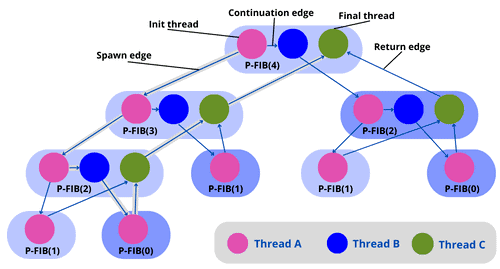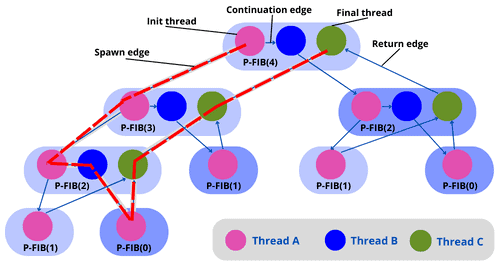## 1. Overview

In computer science, there are various parallel algorithms that can run on a multiprocessor computer, such as multithreaded algorithms.

In this tutorial, we’ll take a look at what this approach means. Then, we’ll use an example to understand it in a better way.

## 2. Concepts of Dynamic Multithreading

Multithreading is a crucial topic for modern computing. There exist many competing models of parallel computation that are essentially different. For example, one can have shared or distributed memory. Since multicore processors are ubiquitous, we focus on a parallel computing model with shared memory.

### 2.1. Dynamic and Static Multithreading

Static threading means that the programmer needs to specify how many processors to use at each point in advance. When it comes to evolving conditions, this can be inflexible.

In dynamic multithreading models, the programmer needs to specify opportunities for parallelism and a concurrency platform manages the decisions of mapping these opportunities to actual static threads.

### 2.2. Concurrency Constructs

A concurrency platform is a software layer that schedules, manages, and coordinates parallel-computing resources. We’ll use a simple extension of the serial programming model that reflects current parallel-computing practice:

• Spawn: Instead of waiting for the child to finish, the procedure instance that executes the spawn (the parent) may continue to execute in parallel with the spawned subroutine (the child)
• Sync: the procedure must wait for the completion of all of its spawning children. It is used when one cannot proceed without pending results
• Parallel: to indicate that each iteration can be done in parallel, we use a loop construct such as “for”

The “parallel” and “spawn” keywords do not impose parallelism. They just indicate that it is possible. This is known as logical parallelism. So, when parallelism is used, we respect “sync”. Every procedure has an implicit “sync” at the end for safety.

## 3. Example: Parallel Fibonacci

Let’s take a slow algorithm and make it parallel. Here is the definition of Fibonacci numbers:Here’s an algorithm (non-parallel) for computing Fibonacci numbers based on the above definition:Here’s the recursion tree of the algorithm cited above:Let’s see what improvement we can get by computing the two recursive calls in parallel using the concurrency keywords:Let’s now look at a formal model for describing parallel computations.

### 4.1. Computation DAG

A computation DAG (directed acyclic graph) will be used to model a multithreaded computation:

• Vertices: vertices () in the graph represent the instructions. To simplify, let’s think of each vertex as a strand (sequence of instructions containing no parallel control, such as sync, spawn, parallel, return from spawn)
• Edges: edges () represent the dependencies between strands or instructions. An edgein () indicates that instruction () must be executed before instruction ()

If there’s an edge between thread (a strand of maximal length will be called a thread)andthey are logically in series; otherwise, they are logically parallel.

### 4.2. Edge Classification

Here’s a categorization of the edges:

• Continuation edge: connects a threadto its successorwithin the same procedure instance
• Spawn edge:is called a spawn edge when a threadspawns a new threadin parallel
• Return edge: when a threadreturns to its calling process andis the thread following the parallel control, the graph includes the return edge### 4.3. Visualization of the Model

Before moving forward to the model for the computation DAG, let’s take a look at the parallel algorithm to compute Fibonacci numbers using threads:Here’s the model for the computation DAG for P-FIB():As we can see in the figure above, the purple thread in the block numberspawns another purple thread in the block number. Then the numberpoints to the purple thread in the block number. The numberpoints to the purple thread in the block number. Then we point to the green thread in the block numberusing the return edge. We continue pointing to the green thread in block numberanduntil we reach the final thread.

Let’s walk through some measures and observations the characterizes dynamic multithreading.

### 5.1. Work

The work of a multithreaded computation is the total time required to execute the entire computation on one processor. So, the work represents the sum of the time taken by each thread.

An ideal parallel computer withprocessors can perform up tounits of work in a one-time step. In other words, it can do up towork intime (is the running time of an algorithm onprocessors). Since the total work is,. Hence, the work law is.

### 5.2. Span

The span is the longest time it takes to execute threads along any path of the computational DAG.

An ideal parallel computer withprocessors cannot run faster than a machine with an infinite number of processors. A computer with an infinite number of processors, on the other hand, can imitate aprocessor machine by employing onlyof its processors. Therefore,, which is known as the span law.

### 5.3. Speedup and Parallelism

The speedup of a computation onprocessors is. So, this ratio defines how much speedup we get with theprocessor as compared to one. Using the work law (), we can conclude that an ideal parallel computer cannot have any more speedup than the number of processors.

The parallelism of a multithreaded computation is. So, this ratio means the average amount of work that can be performed for each step of parallel execution time.

### 5.4. Slackness

The parallel slackness of a multithreaded calculation performed on an ideal parallel computer withprocessors is defined as the parallelism byratio:### 5.5. Performance Measure Example

Let’s use the Fibonacci example (P-FIB()). In the graph below, we usethreads (vertices). As we can see, there arethreads on the longest path (red line in the graph).Let’s assume the unit time for each thread:

• Work:time units
• Span:time units

Let’s now take a look at the work and span of the Fibonacci computation (using the parallel algorithm above of P-FIB()) to compute the parallelism:

• The workis simple since it computes the execution time of the serialized algorithm:• We know that the spanis the longest path in the computational DAG. So, P-FIB() spawns: P-FIB() and P-FIB(). Then, here’s the longest path:• Here’s the parallelism of the P-FIB computation:Let’s now use an example to understand the multithreading algorithm and its operations (parallel, spawn, sync).

### 6.1. Multithreaded Matrix Multiplication Algorithm

Here’s an algorithm for multithreaded matrix multiplication, using thealgorithm:In this algorithm, the longest path is when spawning the outer and inner parallel loop executions, and then theexecutions of the innermost for loop. So, the span of this algorithm is. Hence, the parallelism is.

### 6.2. Multithreaded Merge Sort Algorithm

We employ divide and conquer algorithms for parallelism because they divide the problem into independent subproblems that can be addressed individually. Let’s take a look at merge sort:As we can see, the dividing is in the main procedure Merge-Sort, then we parallelize it by using spawn on the first recursive call. Merge remains a serial algorithm, so its work and span areas before.

Here’s the recurrence for the workof Merge-Sort (it’s the same as the serial version):The recurrence for the spanof Merge-Sort is based on the fact that the recursive calls run in parallel:Here’s the parallelism:As we can see, this is low parallelism, which means that even with massive input, having hundreds of processors would not be beneficial. So, to increase the parallelism, we can speed up the serial Merge.